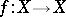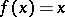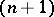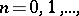# Retract of a topological space

(diff) ← Older revision | Latest revision (diff) | Newer revision → (diff)
A subspaceof a topological spacefor which there is a retraction ofonto. Ifis a Hausdorff space, then every retract ofis closed in. Every non-empty closed subset of the Cantor perfect set is a retract of it. In the transition from a space to a retract of it many important properties are preserved. In particular, every property which is preserved under transition to a continuous image is, like any property of Hausdorff spaces inherited by closed subspaces, stable under passage to a retract. As a result, compactness, connectedness, path-connectedness, separability, an upper bound on the dimension, paracompactness, normality, local compactness, and local connectedness are preserved under passage to a retract. At the same time, a retract of a space may have a simpler structure than the space itself, and be more amenable and convenient for specific research. Thus, a one-point set is a retract of an interval, of a straight line, of a plane, etc. If the spacehas the fixed point property, i.e. if for each continuous transformationthere is a pointsuch that, then each retract ofpossesses the fixed point property too. In particular, the-dimensional sphere is not a retract of the-dimensional ball of a Euclidean space, wheresince the closed ball has the fixed point property (Brouwer's fixed-point theorem), and the sphere does not. A subspaceof a spaceis called a neighbourhood retract of this space if there is inan open subspace which containsand of whichis a retract. The concept of a retract is directly related to the problem of the extension of continuous mappings. Thus, a subspaceis a retract ofif and only if every continuous mapping ofinto an arbitrary topological spacecan be extended to a continuous mapping of the entire spaceinto.
A metric spaceis called an absolute retract (absolute neighbourhood retract) if it is a retract (neighbourhood retract) of every metric space containingas a closed subspace. For a metric spaceto be an absolute retract it is necessary that it be a retract of some convex subspace of a normed linear space, and it is sufficient thatbe a retract of a convex subspace of a locally convex linear space.
Thus, all convex subspaces of locally convex linear spaces are absolute retracts; such is the case, in particular, with a point, an interval, a ball, a straight line, etc. This characterization means that absolute retracts have the following properties. Every retract of an absolute retract is again an absolute retract. Each absolute retract is contractible in itself and is locally contractible. All homology, cohomology, homotopy, and cohomotopy groups of an absolute retract are trivial. A metric spaceis an absolute retract if and only if, given any metric space, a closed subspaceofand a continuous mapping ofinto, the mapping can be extended to a continuous mapping of the entire spaceinto. Absolute neighbourhood retracts are characterized as retracts of open subsets of convex subspaces of normed linear spaces. They include all compact polyhedra. An important property of them is their local contractibility.
If a retraction of a spaceinto a subspaceofis homotopic to the identity mapping ofinto itself, thenis called a deformation retract of. A deformation retract of a spaceis homotopy equivalent to, i.e. they have the same homotopy type. Conversely, two homotopy-equivalent spaces can always be imbedded in a third space in such a way that they are both deformation retracts of this space.